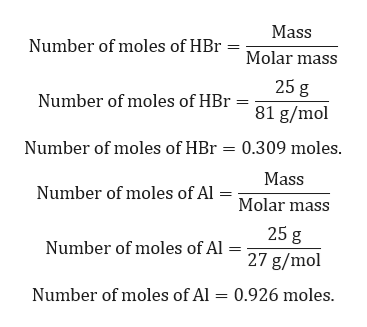# starting with 25.0g of each reactant, how many grams of each product could be produced, and how many grams of each reactant are left over?hydrobromic acid reacts with aluminum metal to produce hydrogen gas and aluminum bromide

Question
2 views

starting with 25.0g of each reactant, how many grams of each product could be produced, and how many grams of each reactant are left over?

hydrobromic acid reacts with aluminum metal to produce hydrogen gas and aluminum bromide

check_circle

Step 1

Given:

Mass of HBr = 25 g.

Molar mass of HBr = 81 g/mol.

Mass of Al = 25 g.

Molar mass of Al = 27 g/mol.

Balanced chemical reaction is given by:

Step 2

Calculation for moles of HBr and Al:help_outlineImage TranscriptioncloseMass Number of moles of HBr Molar mass 25 g Number of moles of HBr = 81 g/mol Number of moles of HBr = 0.309 moles. Mass Number of moles of Al = Molar mass 25 g Number of moles of Al = 27 g/mol Number of moles of Al = 0.926 moles. fullscreen
Step 3

Amount of AlBr3 and H2 produce will depend on limiting reactant.

Moles of HBr = 0.309/6 = 0.0515 moles.

Mo...

### Want to see the full answer?

See Solution

#### Want to see this answer and more?

Solutions are written by subject experts who are available 24/7. Questions are typically answered within 1 hour.*

See Solution
*Response times may vary by subject and question.
Tagged in

### Chemistry